home | O'Reilly's CD bookshelfs | FreeBSD | Linux | Cisco | Cisco Exam

### 4.7 Shift Operators

The shift operators in Java are used for left shift (<<), right shift (>>), and unsigned right shift (>>>) operations. The shift operators may appear in a shift expression: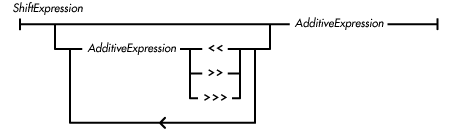The shift operators are equal in precedence and are evaluated from left to right.

References Additive Operators; Order of Operations

### Left Shift Operator <<

The left shift operator << produces a pure value that is its left operand left-shifted by the number of bits specified by its right operand. The << operator may appear in a shift expression. The left shift operator never throws an exception.

Here are some examples of the left shift operator:

```(3<<2) == 12
(-3<<2) == -12
(0x01234567<<4) == 0x12345670
(0xF1234567<<4) == 0x12345670
```

The type of each operand of the left shift operator must be an integer data type, or a compile-time error occurs. The << operator may perform type conversions on its operands; unlike arithmetic binary operators, each operand is converted independently. If the type of an operand is byte, short, or char, that operand is converted to an int before the value of the operator is computed. The type of the value produced by the left shift operator is the type of its left operand.

If the converted type of the left operand is int, only the five least significant bits of the value of the right operand are used as the shift distance. Therefore, the shift distance is in the range 0 through 31. In this case, the value produced by r << s is mathematically equivalent to: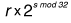If the type of the left operand is long, only the six least significant bits of the value of the right operand are used as the shift distance. Therefore, the shift distance is in the range 0 through 63. In this case, the value produced by r << s is mathematically equivalent to: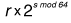References Integer types

### Right Shift Operator >>

The right shift operator >> produces a pure value that is its left operand right-shifted with sign extension by the number of bits specified by its right operand. Right-shifting with sign extension means that shifting a value n places to the right causes the n high order bits to contain the same value as the sign bit of the unshifted value. The >> operator may appear as part of a shift expression. The right shift operator never throws an exception. Here are some examples of the right shift operator:

```(0x01234567>>4) == 0x00123456
(0xF1234567>>4) == 0xFF123456
```

The type of each operand of the right shift operator must be an integer data type, or a compile-time error occurs. The >> operator may perform type conversions on its operands; unlike arithmetic binary operators, each operand is converted independently. If the type of an operand is byte, short, or char, that operand is converted to an int before the value of the operator is computed. The type of the value produced by the right shift operator is the type of its left operand.

If the converted type of the left operand is int, only the five least significant bits of the value of the right operand are used as the shift distance. Therefore, the shift distance is in the range 0 through 31.

In this case, the value produced by r >> s is mathematically equivalent to: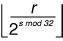The notation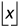means the greatest integer less than or equal to x ; this is called the floor operation.

If the type of the left operand is long, only the six least significant bits of the value of the right operand are used as the shift distance. Therefore, the shift distance is in the range 0 through 63. In this case, the value produced by r >> s is mathematically equivalent to: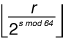References Integer types

### Unsigned Right Shift Operator >>>

The unsigned right shift operator >>> produces a pure value that is its left operand right-shifted with zero extension by the number of bits specified by its right operand. Right-shifting with zero extension means that shifting a value n places to the right causes the n high order bits to contain zero. The >>> operator may appear as part of a shift expression. The unsigned right shift operator never throws an exception.

Here are some examples of the unsigned right shift operator:

```(0x01234567>>>4) == 0x00123456
(0xF1234567>>>4) == 0x0F123456
```

The type of each operand of the unsigned right shift operator must be an integer data type, or a compile-time error occurs. The >>> operator may perform type conversions on its operands; unlike arithmetic binary operators, each operand is converted independently. If the type of an operand is byte, short, or char, that operand is converted to an int before the value of the operator is computed. The type of the value produced by the unsigned right shift operator is the type of its left operand. If the converted type of the left operand is int, only the five least significant bits of the value of the right operand are used as the shift distance. So, the shift distance is in the range 0 through 31. Here, the value produced by r >>> s is the same as:

```s==0 ? r : (r >> s) & ~(-1<<(32-s))
```

If the type of the left operand is long, then only the six least significant bits of the value of the right operand are used as the shift distance. So, the shift distance is in the range 0 through 63. Here, the value produced by r >>> s is the same as the following:

```s==0 ? r : (r >> s) & ~(-1<<(64-s))
```

References Integer typesAdditive OperatorsRelational Comparison Operators